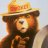# Convert Williams ProGo to ThinkorSwim?

A

#### anty

##### New member
Larry Williams has a professional vs public buying called ProGo. Would anyone be able to convert the TradeStation code below:

Code:
``````PRO GO I:

var: prof(0),pub(0);

Prof=Average(O - C,7);

Value1 = (((Summation( Prof - Lowest(Prof,7),7)) / Summation((highest(Prof,7) - Lowest(Prof,7)),7)))*100;

Pub=Average(O - C,7);

Value2 = (((Summation( Pub - Lowest(Pub,7),7)) / Summation((highest(Pub,7) - Lowest(Pub,7)),7)))*100;

Plot1( Value1, "Prof" ) ;

Plot2( Value2, "Public" ) ;

Plot3( 25, "25" ) ;

Plot4( 75, "75" ) ;

PRO GO II:

var: prof(0),pub(0);

Prof=XAverage(O - C,14);

Pub=XAverage(O - C,14);

Plot1( Prof, "Prof" ) ;

Plot2( Pub, "Public" ) ;

Plot3( 0, "0" ) ;

PRO GO III:

Input: Period (21);

var: prof(0),pub(0);

Prof=XAverage(O - C,Periode)*200;

Pub=XAverage(O - C,Periode)*200;

Plot1( Prof, "Prof" ) ;

Plot2( Pub, "Public" ) ;

Plot3( 0, "0" ) ;``````

Last edited by a moderator:#### horserider

##### Well-known member
VIP
@anty Here you go.

Code:
``````#Larry Williams Pro Go
#coded by DVRL, Optntdr13, daryanlenzatgmaildotcom from tradestation code
#V1:  02/19/2011
#
#
#

declare lower;
#hint Length: Number of periods to average the data.
Input Length = 14;
input movingAverageType = {default "Simple MA", "Exponential MA", "Wilders Smoothing", "Weighted MA", "Hull MA", "Adaptive MA", "Triangular MA", "Variable MA", "Dema MA", "Tema MA"};
input displace = 0;

def Pros = Close - Open;
def Public = Close - Open;

plot X;
plot Y;
switch (movingAverageType)  {
case "Simple MA":
X = Average(Pros[-displace],Length);
Y = Average(Public[-displace],Length);
case "Exponential MA":
X = ExpAverage(Pros[-displace], Length);
Y = ExpAverage(Public[-displace],Length);
case "Wilders Smoothing":
Y = WildersAverage(Public[-displace],Length);
case "Weighted MA":
X = wma(Pros[-displace], Length);
Y = wma(Public[-displace],Length);
case "Hull MA":
X = hullMovingAvg(Pros[-displace], Length);
Y = hullMovingAvg(Public[-displace],Length);
case "Triangular MA":
X = MovAvgTriangular(Pros[-displace], Length);
Y = MovAvgTriangular(Public[-displace],Length);
case "Variable MA":
X = VariableMA(Pros[-displace], Length);
Y = VariableMA(Public[-displace],Length);
case "Dema MA":
X = DEMA(Pros[-displace], Length);
Y = DEMA(Public[-displace],Length);
case "Tema MA":
X = Tema(Pros[-displace], Length);
Y = Tema(Public[-displace],Length);
}

x.SetDefaultColor(CreateColor(188, 0, 240));
y.SetDefaultColor(CreateColor(29,239,255));
def ProsBuying = if x > y then x else y;
def ProsSelling = x < y;

plot zerobase = 0;``````

•SolidChiken and BenTen
S

#### San

##### Member
2019 Donor
@horserider, Do you have Williams' Fractals indicator script..

A

#### anty

##### New member
@anty Here you go.

Code:
``````#Larry Williams Pro Go
#coded by DVRL, Optntdr13, daryanlenzatgmaildotcom from tradestation code
#V1:  02/19/2011
#
#
#

declare lower;
#hint Length: Number of periods to average the data.
Input Length = 14;
input movingAverageType = {default "Simple MA", "Exponential MA", "Wilders Smoothing", "Weighted MA", "Hull MA", "Adaptive MA", "Triangular MA", "Variable MA", "Dema MA", "Tema MA"};
input displace = 0;

def Pros = Close - Open;
def Public = Close - Open;

plot X;
plot Y;
switch (movingAverageType)  {
case "Simple MA":
X = Average(Pros[-displace],Length);
Y = Average(Public[-displace],Length);
case "Exponential MA":
X = ExpAverage(Pros[-displace], Length);
Y = ExpAverage(Public[-displace],Length);
case "Wilders Smoothing":
Y = WildersAverage(Public[-displace],Length);
case "Weighted MA":
X = wma(Pros[-displace], Length);
Y = wma(Public[-displace],Length);
case "Hull MA":
X = hullMovingAvg(Pros[-displace], Length);
Y = hullMovingAvg(Public[-displace],Length);
case "Triangular MA":
X = MovAvgTriangular(Pros[-displace], Length);
Y = MovAvgTriangular(Public[-displace],Length);
case "Variable MA":
X = VariableMA(Pros[-displace], Length);
Y = VariableMA(Public[-displace],Length);
case "Dema MA":
X = DEMA(Pros[-displace], Length);
Y = DEMA(Public[-displace],Length);
case "Tema MA":
X = Tema(Pros[-displace], Length);
Y = Tema(Public[-displace],Length);
}

x.SetDefaultColor(CreateColor(188, 0, 240));
y.SetDefaultColor(CreateColor(29,239,255));
def ProsBuying = if x > y then x else y;
def ProsSelling = x < y;

plot zerobase = 0;``````

A

#### anty

##### New member
Wow, awesome @horserider ! Where did you find that? I searched all over the thinkscript cloud for that. Thanks!#### horserider

##### Well-known member
VIP
@anty No idea now. Just had it in my list of studies.

A

#### anty

##### New member
@anty No idea now. Just had it in my list of studies.
Cool. Thanks!How do I convert this ATR% chart study into a scanner watchlist column? Questions 5